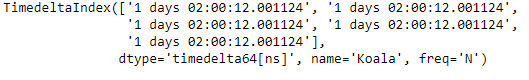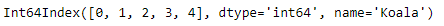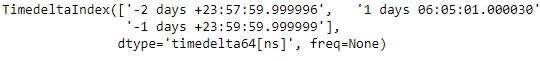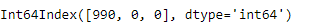# Python | Pandas TimedeltaIndex.nanoseconds

Python is a great language for doing data analysis, primarily because of the fantastic ecosystem of data-centric python packages. Pandas is one of those packages and makes importing and analyzing data much easier.

Pandas` TimedeltaIndex.nanoseconds` attribute return the number of nanoseconds for each element in the TimedeltaIndex object. The value returned ranges from 0 to less than 1 microsecond.

Syntax : TimedeltaIndex.nanoseconds

Return : index object

Example #1: Use `TimedeltaIndex.nanoseconds` attribute to find out the nanoseconds value for each entry of the TimedeltaIndex object.

 `# importing pandas as pd ` `import` `pandas as pd ` ` `  `# Create the TimedeltaIndex object ` `tidx ``=` `pd.TimedeltaIndex(start ``=``'1 days 02:00:12.001124'``, periods ``=` `5``,  ` `                                            ``freq ``=``'N'``, name ``=``'Koala'``) ` ` `  `# Print the TimedeltaIndex ` `print``(tidx) `

Output :Now we will print the number of nanoseconds in each entry of the object.

 `# find nanoseconds number ` `tidx.nanoseconds `

Output :As we can see in the output, the `TimedeltaIndex.nanoseconds` attribute has returned the number of nanoseconds in each element of the object.

Example #2: Use `TimedeltaIndex.nanoseconds` attribute to find out the nanoseconds value for each entry of the TimedeltaIndex object.

 `# importing pandas as pd ` `import` `pandas as pd ` ` `  `# Create the TimedeltaIndex object ` `tidx ``=` `pd.TimedeltaIndex(data ``=``[``'-1 days 2 min 3us 10ns'``, ``'1 days 06:05:01.000030'``,  ` `                                                     ``'-1 days + 23:59:59.999999'``]) ` ` `  `# Print the TimedeltaIndex ` `print``(tidx) `

Output :Now we will print the number of nanoseconds in each entry of the object.

 `# find nanoseconds number ` `tidx.nanoseconds `

Output :As we can see in the output, the `TimedeltaIndex.nanoseconds` attribute has returned the number of nanoseconds in each element of the object.

My Personal Notes arrow_drop_upCheck out this Author's contributed articles.

If you like GeeksforGeeks and would like to contribute, you can also write an article using contribute.geeksforgeeks.org or mail your article to contribute@geeksforgeeks.org. See your article appearing on the GeeksforGeeks main page and help other Geeks.

Please Improve this article if you find anything incorrect by clicking on the "Improve Article" button below.

Article Tags :

Be the First to upvote.

Please write to us at contribute@geeksforgeeks.org to report any issue with the above content.